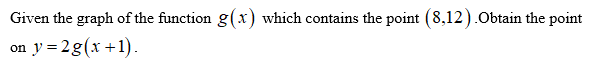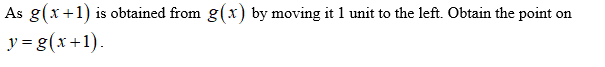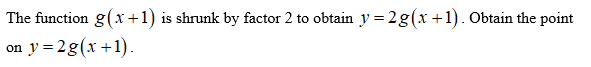# The graph of the function g(x) contains the point (8,12). What point must be on the graph of y=2g(x+1)?y=2g(x+1) contains the point

Question

The graph of the function g(x) contains the point (8,12). What point must be on the graph of y=2g(x+1)?

y=2g(x+1) contains the point

check_circleExpert Solution
Step 1Step 2Step 3...

### Want to see the full answer?

See Solution

#### Want to see this answer and more?

Solutions are written by subject experts who are available 24/7. Questions are typically answered within 1 hour*

See Solution
*Response times may vary by subject and question
Tagged in

### Other